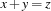# The Mozart Constraint Extensions Tutorial

## Tobias Müller

This tutorial provides the knowledge to go beyond the built-in constraint capabilities of Mozart Oz 3. This tutorial is complemented by ``The Mozart Constraint Extensions Reference''.

Motivation

A major design goal of Oz is to provide for a wide range of applications the right level of programming abstractions. Though Mozart Oz 3 features a full-fledged finite domain and finite set constraint solver providing for the functionality required to solve combinatorial problems efficiently, it is often desirable to implement constraints in C++. There may be several reasons to do so, as for example, that a given algorithm requires destructively updateable data structures or an already existing C++ library shall be used. Consequently, we opened the constraint solver of Mozart Oz 3 by adding a C/C++ interface for implementing so-called constraint propagators. Hereby, a constraint propagator is the implementation of a constraint. Further, it may be desirable to have a constraint system available that is not provided by Mozart Oz 3 and one wants to implement it from scratch. Even such cases can be handled by the C/C++ interface. Finally, the integration of linear (integer) programming solvers is explained which are standard means in Operations Research to tackle certain combinatorial problem classes.

Structure of the Manual

The user manual consists of three parts:

1. The first part explains how to implement various propagators. It starts with a propagator for the constraintover finite domains and introduces the tools and techniques needed. This propagator will be refined such that it is able to detect equal variables and reduces to a more specialised propagator. Then a functionally nestable version of the addition propagator will be derived. We go on with a propagator that can deal with vectors of variables. As example serves the so-called element constraint. The implementation of a propagator using finite set and finite domain constraints is explained next. Finally more advanced topics, like the implementation of reified constraints, are discussed.

Note that it is not the intention of this manual to provide sophisticated algorithms.

2. The second part explains the implementation of constraint systems from scratch, i. e., not only the propagators of a certain constraint system but also the basic constraints. As example, constraints over real intervals are implemented.

3. The third part explains the integration and usage of linear programming solvers like CPLEX [ILO97] and LP_SOLVE [Har] from within Mozart Oz 3. To demonstrate the benefits of jointly using propagation-based and linear programming-based solvers knapsack problems are tackled.

Prerequisites

The reader is supposed to have a working knowledge in the C/C++ programming language and to be familiar with constraint-based problem solving techniques in Oz. An excellent supplementary text book on C++ is [Mur93]. Constraint-based problem solving techniques in Oz are explained in ``Finite Domain Constraint Programming in Oz. A Tutorial.'' resp. ``Problem Solving with Finite Set Constraints in Oz. A Tutorial.''. The CPI uses the native functor interface of Mozart Oz 3. Have a look at Part VI of ``Application Programming'' resp. ``Interfacing to C and C++'' for details.

Tobias Müller
Version 1.4.0 (20080702)